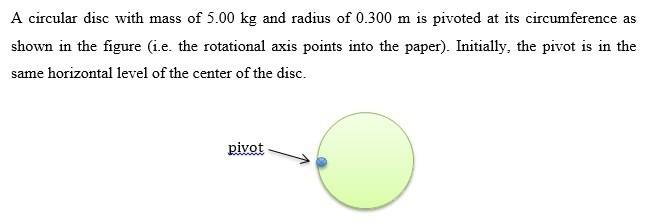# Moment of inertia and angular velocity

nomorenomore

## Homework Statement(a) Calculate the moment of inertia I of the disc when it rotates about the pivot as shown in the figure.
(b) If the disc is released from rest, determine the angular speed, ω, of the disc at its lowest point.

## Homework Equations

a) Id = Icm + md^2
Icm = 1/2*M*R^2
b) a = r*γ, a=g
(ωf)^2 = (ωi)^2 + 2*γ*θ

## The Attempt at a Solution

a) Id = Icm + md^2
= 1/2MR^2 + 5(0.3^2)
= 1/2*5*0.3^2 + 5(0.3^2)
= 0.675 kg/m^2

b) a = r*γ, a=g, γ = g/r
θ = ¼*2pi
(ωf)^2 = (ωi)^2 + 2*γ*θ
ωf = sqrt(2*g/r*1/4*2pi) = sqrt(2*9.8/0.3*1/4*2pi = 10.1 rad/s

Am I doing the question correctly?
Thank you very much.

## Answers and Replies

voko
I am not sure what the "relevant equation" for (b) means.

nomorenomore
I am not sure what the "relevant equation" for (b) means.

b) a = r*γ, a=g
(ωf)^2 = (ωi)^2 + 2*γ*θ

Oh, I'm sorry for this.
I was trying to show that

linear acceleration = radius * angular acceleration,
where linear acceleration = gravitational acceleration, in this case

(final angular velocity)^2 = (initial angular velocity)^2 + 2*angular acceleration*angular displacement

voko
Don't you think angular acceleration should depend on the moment of inertia?

Besides, why do you think it will be constant?